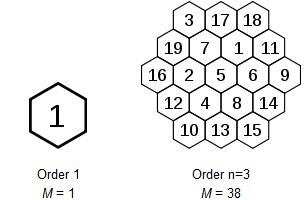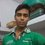# Magic HexagonMost of us are familiar with Magic Squares and its wonders. But little is known about Magic Hexagons. This post is attributed for describing "Magic Hexagons".

A magic hexagon of order n is an arrangement of numbers in a centered hexagonal pattern with n cells on each edge, in such a way that the numbers in each row, in all three directions, sum to the same magic constant. A normal magic hexagon contains the consecutive integers from 1 to 3n^2 − 3n + 1. It turns out that normal magic hexagons exist only for n = 1 (which is trivial) and n = 3. Moreover, the solution of order 3 is essentially unique. These hexagons are shown for your reference. The first magic hexagon has Magic sum 1 and second has Magic Sum 38.Note by Shivanand Pandey
7 years, 4 months ago

This discussion board is a place to discuss our Daily Challenges and the math and science related to those challenges. Explanations are more than just a solution — they should explain the steps and thinking strategies that you used to obtain the solution. Comments should further the discussion of math and science.

When posting on Brilliant:

• Use the emojis to react to an explanation, whether you're congratulating a job well done , or just really confused .
• Ask specific questions about the challenge or the steps in somebody's explanation. Well-posed questions can add a lot to the discussion, but posting "I don't understand!" doesn't help anyone.
• Try to contribute something new to the discussion, whether it is an extension, generalization or other idea related to the challenge.

MarkdownAppears as
*italics* or _italics_ italics
**bold** or __bold__ bold
- bulleted- list
• bulleted
• list
1. numbered2. list
1. numbered
2. list
Note: you must add a full line of space before and after lists for them to show up correctly
paragraph 1paragraph 2

paragraph 1

paragraph 2

[example link](https://brilliant.org)example link
> This is a quote
This is a quote
    # I indented these lines
# 4 spaces, and now they show
# up as a code block.

print "hello world"
# I indented these lines
# 4 spaces, and now they show
# up as a code block.

print "hello world"
MathAppears as
Remember to wrap math in $$ ... $$ or $ ... $ to ensure proper formatting.
2 \times 3 $2 \times 3$
2^{34} $2^{34}$
a_{i-1} $a_{i-1}$
\frac{2}{3} $\frac{2}{3}$
\sqrt{2} $\sqrt{2}$
\sum_{i=1}^3 $\sum_{i=1}^3$
\sin \theta $\sin \theta$
\boxed{123} $\boxed{123}$

Sort by:

This is interesting. Do you know why no other magic hexagon of higher orders exist?

With $n=2$, why can't we have

$\begin{array} { l l l l } & 1 & & 2 \\ 5 & & 4 & & 3 \\ & 6 & & 7 \\ \end{array}$

The entries in each row sum to 12. Is there something that I'm missing?

Staff - 7 years, 4 months ago

I believe what you wrote down isn't exactly correct. Although a few of the rows sum to 12, not all of them do. I'll go through a few of the rows.

$1 + 2 \neq 12$

$5 + 6 \neq 12$

$6 + 7 \neq 12$

and so on.

- 7 years, 4 months ago

Ah, even 'partial' rows count. That makes sense now. Thanks!

Staff - 7 years, 4 months ago

Higher order magic hexagons do exist, but cannot be 'normal'.

- 7 years, 4 months ago